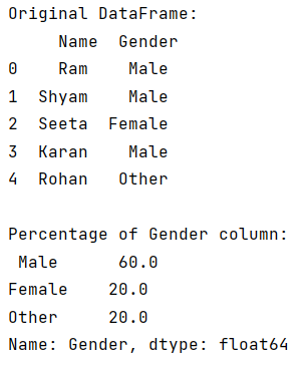# Pandas get frequency of item occurrences in a column as percentage

Given a Pandas DataFrame, we need to get the frequency of item occurrences in a column as percentage.
Submitted by Pranit Sharma, on July 26, 2022

Pandas is a special tool that allows us to perform complex manipulations of data effectively and efficiently. Inside pandas, we mostly deal with a dataset in the form of DataFrame. DataFrames are 2-dimensional data structures in pandas. DataFrames consist of rows, columns, and data.

Pandas consist of almost every kind of mathematical and logical function which helps us to perform tough and long calculations with no effort.

To fetch the frequency of item occurrences in a separate column as a percentage, we will use the value_count() method and find the percentage for each item.

We will first use value_count which will return the count of total occurrences of each value and then we will divide each value by the total length of the dataFrame.

Finally, we will multiply this value by 100 to get the percentage.

Let us understand with the help of an example,

## Python code to get frequency of item occurrences in a column as percentage

```# Importing pandas package
import pandas as pd

# Creating a Dictionary
d = {
'Name':['Ram','Shyam','Seeta','Karan','Rohan'],
'Gender':['Male','Male','Female','Male','Other']
}

# Creating a DataFrame
df = pd.DataFrame(d)

# Display original DataFrame
print("Original DataFrame:\n",df,"\n")

# Calculating percentage
result = (df['Gender'].value_counts()/len(df)*100)

# Display result
print("Percentage of Gender column:\n",result)
```

Output:Languages: » C » C++ » C++ STL » Java » Data Structure » C#.Net » Android » Kotlin » SQL
Web Technologies: » PHP » Python » JavaScript » CSS » Ajax » Node.js » Web programming/HTML
Solved programs: » C » C++ » DS » Java » C#
Aptitude que. & ans.: » C » C++ » Java » DBMS
Interview que. & ans.: » C » Embedded C » Java » SEO » HR
CS Subjects: » CS Basics » O.S. » Networks » DBMS » Embedded Systems » Cloud Computing
» Machine learning » CS Organizations » Linux » DOS
More: » Articles » Puzzles » News/Updates